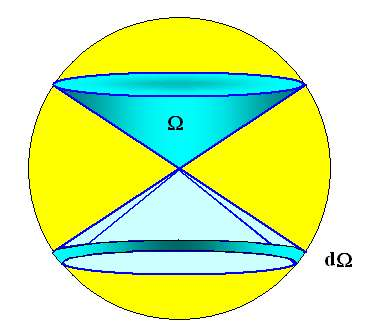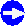Spherical CoordinatesFor many mathematical problems, it is far easier to use spherical coordinates instead of Cartesian ones.In essence, a vector r (we drop the underlining here) with the Cartesian coordinates (x,y,z) is expressed in spherical coordinates by giving its distance from the origin (assumed to be identical for both systems) |r|, and the two angles j and Q between the direction of r and the x- and z-axis of the Cartesian system.This sounds more complicated than it actually is: j and Q are nothing but the geographic longitude and latitude. The picture below illustrates this.This is simple enough, for the translation from one system to the other one we have the equations
x  =  r · sinQ · cosj   r  =   (x2 + y2 + z2)½
y  =   r · sinQ · sinj   j  =  arctg (y/x)
z  =   r · cos Q   Q  =   arctg (x2 + y2 + z2 )½
zNot particularly difficult, but not so easy either.Note that there is now a certain ambiguity: You describe the same vector for an ¥ set of values for Q and f, because you always can add n·2p (n = 1,2,3...) to any of the two angles and obtain the same result.This has a first consequence if you do an integration. Lets look at the ubiquitous case of normalizing a wave function y (x,y,z) by demanding that
 y (x,y,z) · dxdydz  =  1 ¥ ó õ –¥ ¥ ó õ –¥ ¥ ó õ –¥In spherical coordinates, we have
 y (r,j,Q) · dr dj dQ  =  1 ¥ ó õ 0 2p ó õ 0 p ó õ 0You no longer integrate from -¥ to ¥ with respect to the angles, but from 0 to 2p for j and from 0 to p for Q because this covers all of space. Notice the different upper bounds!Lets try this by computing the volume VR of a sphere with radius R. This is always done by summing over all the differential volume elements dV inside the body defined by some equationIn Cartesian coordinates we have for the volume element dV = dxdydz, and for the integral:
 ??? dxdydz ¥ ó õ –¥ ¥ ó õ –¥ ¥ ó õ –¥Well, if you can just formulate the integral, let alone solving it, you are already doing well!In spherical coordinates we first have to define the volume element. This is relatively easily done by looking at a drawing of it:An incremental increase in the three coordinates by dr, dj, and dQ produces the volume element dV which is close enough to a rectangular body to render its volume as the product of the length of the three sides.Looking at the basic geometry, the length of the three sides are identified as
dr, r · dQ, and r · sinQ · dj, which gives the volume element

dV  =  r2 · sinQ · dr · dQ · djThe volume of our sphere thus results from the integral

 Vr  =   r2 · sinQ · dr dj dQ =   2p · r2 · sinQ · dr dQ  =  2p · [–cos p + cos 0] · r2 · dr ¥ ó õ 0 2p ó õ 0 p ó õ 0 ¥ ó õ 0 p ó õ 0 ¥ ó õ 0

Vr  =  2p ·  · 1/3R3  =  (4/3) · p · R3         q.e.d.Not extremely easy, but no problem either.Next, consider differential operators, like div, rot, or more general,and2 (= D).Lets just look at D to see what happens. We have (for some function U)

Cartesian coordinates
D = 2U
+ 2U
+ 2U
x2 y2 z2

Spherical Coordinates
D = 2U
r2
+   2
r
·   U
r
+   1
r2 · sin2 · Q
·   2U
j2
+   1
r2
·   2U
Q2
+   cotg Q
r2
·   U
QLooks messy, OK, but it is still a lot easier to work with this D operator than with its Cartesian counterpart for problems with spherical symmetry; witness the solution of Schrödingers equation for the Hydrogen atom.Looking back now on our treatment of the orientation polarization, we find yet another way of expressing spherical coordinates for problems with particular symmetry:We use a solid angle W and its increment dW.A solid angle W is defined as the ratio of the area on a unit sphere that is cut out by a cone with the solid angle W to the total surface of a unit sphere ( = 4pR2 = = 4p for R = 1).A solid angle of 4p therefore is the same as the total sphere, and a solid angle of p is a cone with a (plane) opening angle of 120o (figure that out our yourself).An incremental change of a solid angle creates a kind of ribbon around the opening of the cone defined by W. This is shown belowRelations with spherical symmetry where the value of Q does not matter - i.e. it does not appear in the relevant equations - are more elegantly expressed with the solid angle W.That is the reason why practically all text books introduce Q in the treatment of the polarization orientation. And in order to be compatible with most text books, that was what we did in the main part of the Hyperscript.Of course, eventually, we have to replace Q and dQ by the basic variables that describe the problem, and that is only the angle d in our problem (same thing as the angle j here).Expressing dQ in terms of d is easy (compare the picture in the main text)The radius ot the circle bounded by the dQ ribbon is r·sind = sind because we have the unit sphere, and its width is simply dd.Its incremental area is thus the relation that we used in the main part.
dQ  =  2p · sind · dd3.2.4 Orientation Polarization

© H. Föll (Electronic Materials - Script)